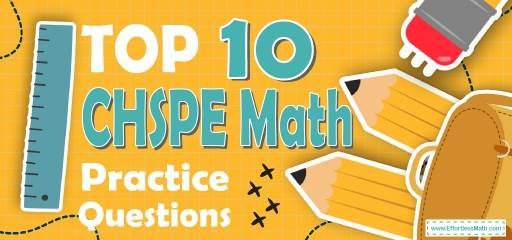# Top 10 CHSPE Math Practice QuestionsPreparing for the CHSPE Math test? The best way to prepare for your CHSPE Math test is to work through as many CHSPE Math practice questions as possible. Here are the top 10 CHSPE Math practice questions to help you review the most important CHSPE Math concepts. These CHSPE Math practice questions are designed to cover mathematics concepts and topics that are found on the actual test. The questions have been fully updated to reflect the latest 2022 CHSPE guidelines. Answers and full explanations are provided at the end of the post.

Start your CHSPE Math test prep journey right now with these sample CHSPE Math questions.

## CHSPE Math Practice Questions

1- What is the value of the expression $$(5(x-2y)+(2-x))^2$$ when$$x=3$$ and $$y=-2$$ ?

A. 20

B. 50

C. 1156

D. 36

2- What is the slope of a line that is perpendicular to the line
$$4x-2y=12$$?

A. $$-2$$

B. 4

C. 2

D.$$-\frac{1}{2}$$

3- Which of the following is equal to the expression below?
$$(2x+2y)(2x-y)$$

A. $$4x^2- 2y^2$$

B. $$2x^2+6xy-2y^2$$

C. $$24x^2+2xy-2y^2$$

D. $$4x^2+2xy-2y^2$$

4- What is the product of all possible values of x in the following equation?
$$|x-10| = 3$$

A. 3

B. 7

C. 13

D. 91

5- $$[6 \times (- 24) + 8] – (-4) + [4 \times 5] \div 2 =$$?

A. 111

B. $$-112$$

C. $$-122$$

D. $$-144$$

6- What is the value of $$x$$ in the following system of equations?
$$2x+5y=11$$
$$4x-2y=-14$$

A. $$-1$$

B. 1

C. 4

D. $$-2$$

7- What is the median of these numbers? 2, 27, 28, 19, 67, 44, 35

A. 19

B. 28

C. 44

D. 35

8- A swimming pool holds 2,000 cubic feet of water. The swimming pool is 25 feet long and 10 feet wide. How deep is the swimming pool?_______

9- The area of a circle is $$64 π$$. Which of the following is the circumference of the circle?

A. 8$$\pi$$

B. 12$$\pi$$

C. 16$$\pi$$

D. 64$$\pi$$

10- What is the value of $$3^6$$ ?______

## Best CHSPE Math Tutor Resource for 2022

1- D
Plug in the value of $$x$$ and $$y$$.
$$x=3$$ and $$y=-2$$
$$(5(x-2y)+(2-x))^2=(5(3-2(-2))+(2-3))^2=(5(3+4)+(-1))^2 = (34)^2=36$$

2- D
The equation of a line in slope intercept form is: $$y=mx+b$$
Solve for y.
$$4x-2y=12 {\Rightarrow} -2y=12-4x {\Rightarrow} y=(12-4x){\div}(-2) {\Rightarrow} y=2x-6$$
The slope of this line is 2.
The product of the slopes of two perpendicular lines is $$-1$$.
Therefore, the slope of a line that is perpendicular to this line is:
$$m_{1} {\times} m_{2} = -1 {\Rightarrow} 2 {\times} m_{2} = -1 {\Rightarrow} m_{2} = \frac{-1}{2}$$

3- D
Use FOIL method.
$$(2x+2y)(2x-y) = 4x^2-2xy+4xy-2y^2=4x^2+2xy-2y^2$$

4- D
To solve absolute values equations, write two equations.
$$x-10$$ could be positive 3, or negative 3. Therefore,
$$x-10=3 \Rightarrow x=13$$
$$x-10=-3 \Rightarrow x=7$$
Find the product of solutions: $$7 \times 13 = 91$$

5- C
Use PEMDAS (order of operation):
$$[6 {\times} (- 24) + 8] – (- 4) + [4 × 5] {\div} 2$$
$$[- 144 + 8] – (- 4) +  {\div} 2 = [- 144 + 8] – (- 4) + 10$$
$$[- 136] – (- 4) + 10 = [- 136] + 4 + 10 = – 122$$

6- D
Solving Systems of Equations by Elimination
Multiply the first equation by $$(-2)$$, then add it to the second equation.
$${-2(2x+5y= 11) \ 4x-2y=-14} {\Rightarrow} {-4x-10y= -22 \ 4x-2y=-14} {\Rightarrow} {-12y= -36} {\Rightarrow} {y= 3}$$
Plug in the value of y into one of the equations and solve for $$x$$.
$$2x+5(3)= 11 {\Rightarrow} 2x+15= 11 {\Rightarrow} 2x= -4 {\Rightarrow} x= -2$$

7- B
Write the numbers in order:
2, 19, 27, 28, 35, 44, 67
Median is the number in the middle. So, the median is 28

8- 8
Use formula of rectangle prism volume.
$$V = (length) (width) (height) {\Rightarrow} 2000 = (25) (10) (height) {\Rightarrow} height = 2000 {\div} 250 = 8$$

9- C
The area of the circle is $$16 π$$. Use the formula of areas of circles.
$$Area = πr^2 ⇒ 64 π> πr^2⇒ 64 > r^2⇒ r < 8$$
The radius of the circle is 8. Let’s put 8 for the radius. Now, use the circumference formula:
$$Circumference =2πr=2π (8)=16π$$

10- 729
$$(3^6 ) = 3 × 3 × 3 × 3 × 3 × 3 = 729$$

Looking for the best resource to help you succeed on the CHSPE Math test?

## The Best Books to Ace the CHSPE Math Test

### What people say about "Top 10 CHSPE Math Practice Questions - Effortless Math: We Help Students Learn to LOVE Mathematics"?

No one replied yet.

X
52% OFF

Limited time only!

Save Over 52%

SAVE $40 It was$76.99 now it is \$36.99